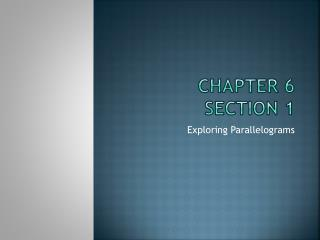DownloadDownload PresentationChapter 6 Section 1

# Chapter 6 Section 1

Download Presentation## Chapter 6 Section 1

- - - - - - - - - - - - - - - - - - - - - - - - - - - E N D - - - - - - - - - - - - - - - - - - - - - - - - - - -
##### Presentation Transcript

1. Chapter 6Section 1 Exploring Parallelograms

2. Warm-Up A B Given: BD is a perpendicular bisector of AC. 1) Can you say that triangle AED is congruent to triangle CED? Yes, by LL 2) Can you say that triangle AED is congruent to triangle CEB? No 3) If m<4 > m<2, what can you say about their opposite segments? BC > CD 4) If m<2 > m<1 and AB is congruent to BC, what can you say about the segments in the triangles? DC > AD E D C B 1 2 3 4 D C A

3. Vocabulary Quadrilateral- A four-sided polygon. Parallelogram- A quadrilateral in which both pairs of opposite sides are parallel. Diagonals- In a polygon, a segment joining nonconsecutive vertices of a polygon.

4. Vocabulary Cont. Theorem 6-1- Opposite sides of a parallelogram are congruent. Theorem 6-2- Opposite angles of a parallelogram are congruent. Theorem 6-3- Consecutive angles in a parallelogram are supplementary. (<1 + <2 = 180, <2 + <3 = 180, <3 + <4 =180, and <4 + <1 = 180)  Theorem 6-4- The diagonals of a parallelogram bisect each other. 1 2 4 3

5. Example 1: WXYZ is a parallelogram, m<ZWX = b, and m<WXY = d. Find the values of a, b, c, and d. Find a: Diagonals bisect each other. 15 = a Find b: Opposite angles are congruent. m<ZWX = m<XYZ b= 31 + 18 b= 49 Find c: Opposite sides are congruent. 22 = 2c 11 = c W 2c 15 X a Z 18 31 22 Y Find d: Consecutive angles are supplementary. 180 = b + d 180 = 49 + d 131 = d

6. Example 2: MATH is a parallelogram. Find the values of w, x, y, and z. Find x: Opposite sides are congruent. 15 = x Find y: Opposite angles are congruent. 55 = y Find z: Alternate interior angles are congruent. y = z 55 = z 15 M A w y 55 z H x T Find w: Consecutive angles are supplementary. 180 = w + y 180 = w + 55 125 = w

7. Example 3: Complete each statement about parallelogram CDFG. Then name the theorem or definition that justifies your answer. A) CH is congruent to ______? HF; Diagonals bisect each other. B) GF is parallel to _______? CD; Opposite sides are parallel. C) <DCG is congruent to _______? <DFG; Opposite angles are congruent. D) DC is congruent to _______? GF; Opposite sides are congruent. C D H G F E) <DCG is supplementary to _________? <CDF and <CGF; Consecutive angles are congruent. F) Triangle HGC is congruent to triangle _____? Triangle HDF; SSS because diagonals bisect each other and opposite sides are congruent.

8. Example 4: In parallelogram ABCD, AB = 2x + 5, m<BAC = 2y, m<B = 120, m<CAD = 21, and CD = 21. Find the values of x and y. Draw a diagram. Find x: Opposite sides are congruent. 21 = 2x + 5 16 = 2x 8 = x Find y: Consecutive interior angles are supplementary. 180 = m<DAB + m<B 180 = m<CAD + m<BAC + m<B 180 = 21 + 2y + 120 180 = 141 + 2y 39 = 2y 19.5 = y 2x + 5 A B 2y 120 21 D 21 C

9. Example 5: Quadrilateral WXYZ is a parallelogram with m<W = 47. Find the measure of angles X, Y, and Z. Draw a diagram. Find m<Y: Opposite angles are congruent. m<W = m<Y 47 = m<Y Find m<X: Consecutive interior angles are supplementary. 180 = m<X + m<W 180 = m<X + 47 133 = m<X Find m<Z: Opposite angles are congruent. m<X = m<Z 133 = m<Z W X Z Y